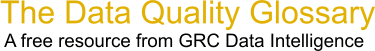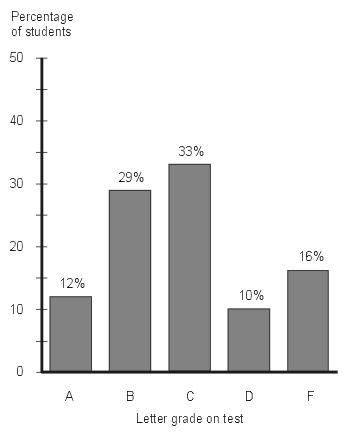Choose Index below for a list of all words and phrases defined in this glossary.

# Bar Chart

index | Index

## Bar Chart - definitions

Bar Chart - A bar chart is a graphical comparison of several quantities in which the lengths of the horizontal or vertical bars represent the relative magnitude of the values.

[Category=Data Quality ]

Source: iSixSigma, 01 January 2011 09:36:10, http://www.isixsigma.com/index.php?option=com_glossaryThese advertisers support this free service

bar graph - A bar graph is a pictorial rendition of statistical data in which the independent variable can attain only certain discrete values. The dependent variable may be discrete or continuous. The most common form of bar graph is the vertical bar graph, also called a column graph.

In a vertical bar graph, values of the independent variable are plotted along a horizontal axis from left to right. Function values are shown as shaded or colored vertical bars of equal thickness extending upward from the horizontal axis to various heights. In a horizontal bar graph, the independent variable is plotted along a vertical axis from the bottom up. Values of the function are shown as shaded or colored horizontal bars of equal thickness extending toward the right, with their left ends vertically aligned.The illustration is a vertical bar graph depicting the results of a test given to a hypothetical class of students. Each letter grade (A through F) is denoted by a gray vertical bar of a certain height. The total of the percentages is equal to 100. (If the total were not 100%, the accuracy of the graph or the supporting data would be suspect!) The percentage of students receiving a specific grade is directly proportional to the height of the bar representing that grade. The bar thickness is chosen only for illustration clarity.

In a specialized type of vertical bar graph called a Pareto chart , values of the dependent variable are plotted in decreasing order of relative frequency from left to right. Another type of bar graph called a histogram uses rectangles to show the frequency of data items in successive numerical intervals of equal size. Other types of bar graphs allow plotting multiple ranges of the dependent variable, multiple independent variables, positive/negative variables and multi-category variables.   [Category=Data Management ]

Source: WhatIs.com, 06 July 2013 09:26:34, http://whatis.techtarget.com/glossary/Data-and-Data-ManagementData Quality Glossary.  A free resource from GRC Data Intelligence. For comments, questions or feedback: dqglossary@grcdi.nl